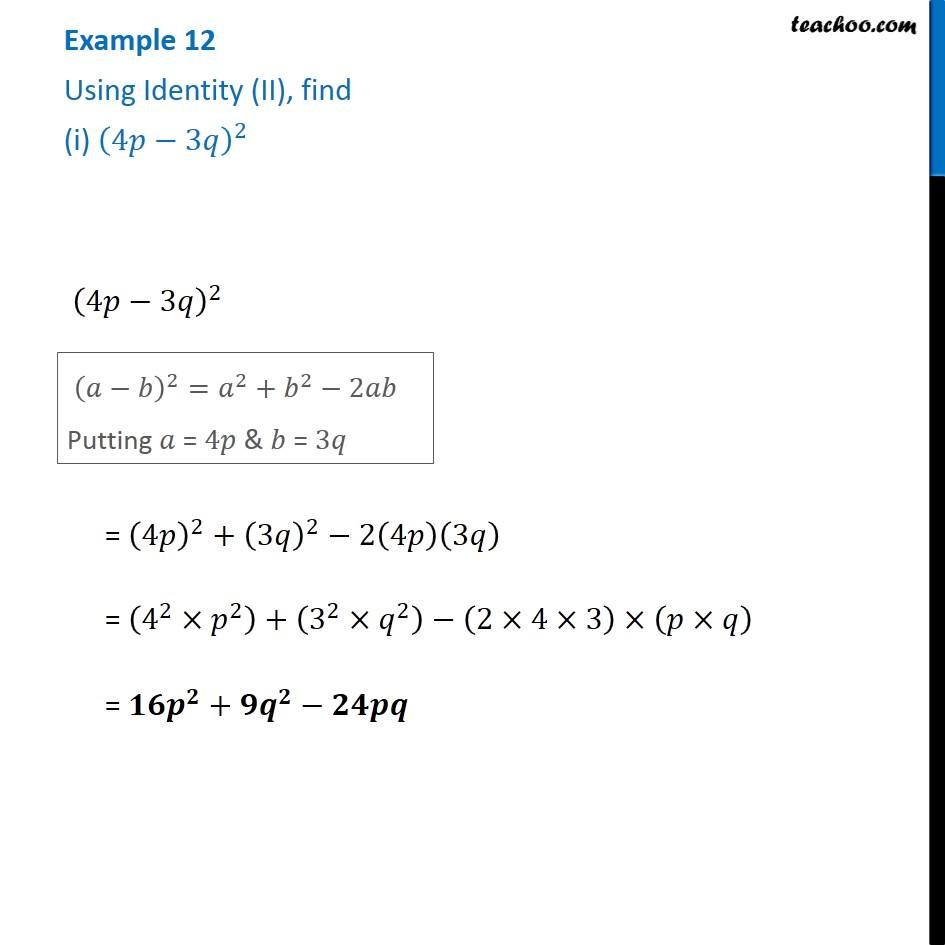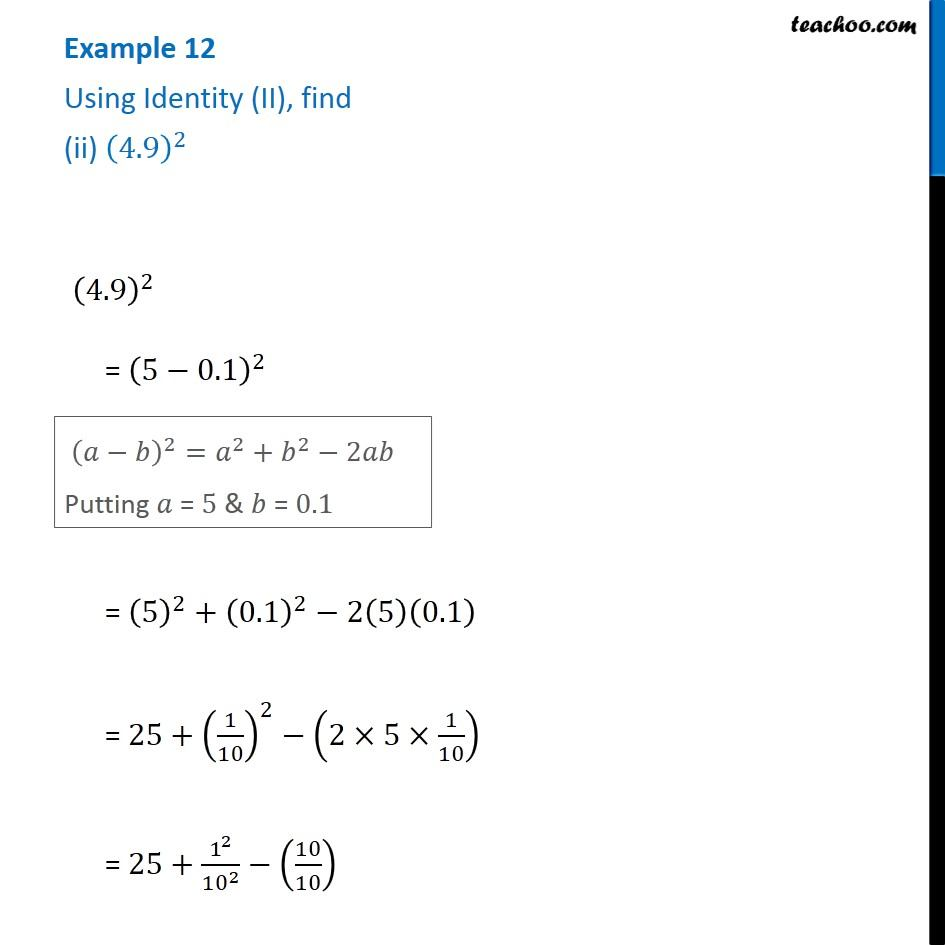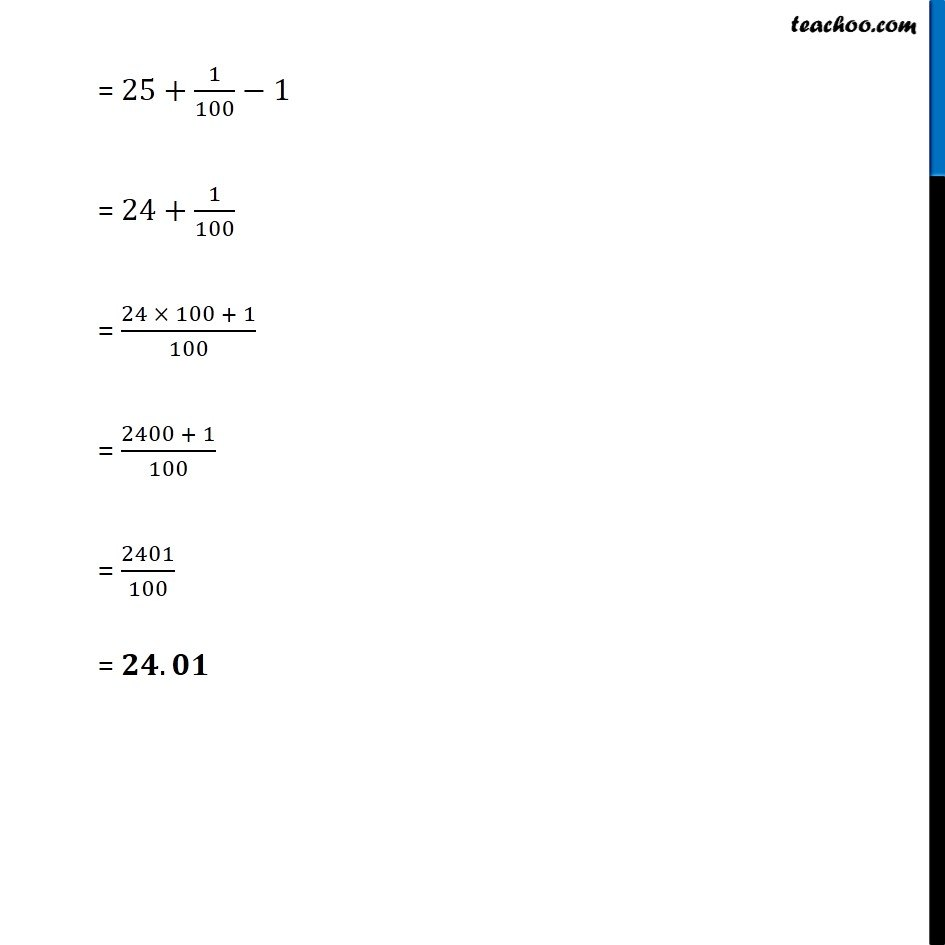1. Chapter 9 Class 8 Algebraic Expressions and Identities
2. Serial order wise
3. Examples

Transcript

Example 12 Using Identity (II), find (i) (4𝑝−3𝑞)^2 (4𝑝−3𝑞)^2 (𝑎−𝑏)^2=𝑎^2+𝑏^2−2𝑎𝑏 Putting 𝑎 = 4𝑝 & 𝑏 = 3𝑞 = (4𝑝)^2+(3𝑞)^2−2(4𝑝)(3𝑞) = (4^2×𝑝^2 )+(3^2×𝑞^2 )−(2×4×3)×(𝑝×𝑞) = 𝟏𝟔𝒑^𝟐+𝟗𝒒^𝟐−𝟐𝟒𝒑𝒒 Example 12 Using Identity (II), find (ii) (4.9)^2 (4.9)^2 = (5−0.1)^2 (𝑎−𝑏)^2=𝑎^2+𝑏^2−2𝑎𝑏 Putting 𝑎 = 5 & 𝑏 = 0.1 = (5)^2+(0.1)^2−2(5)(0.1) = 25+(1/10)^2−(2×5×1/10) = 25+1^2/〖10〗^2 −(10/10) = 25+1/100−1 = 24+1/100 = (24 × 100 + 1)/100 = (2400 + 1)/100 = 2401/100 = 𝟐𝟒.𝟎𝟏

Examples